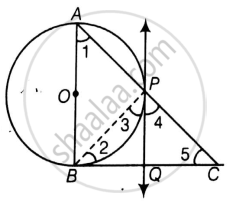# In a right triangle ABC in which ∠B = 90°, a circle is drawn with AB as diameter intersecting the hypotenuse AC and P. Prove that the tangent to the circle at P bisects BC. - Mathematics

Diagram
Sum

In a right triangle ABC in which ∠B = 90°, a circle is drawn with AB as diameter intersecting the hypotenuse AC and P. Prove that the tangent to the circle at P bisects BC.

#### SolutionGiven: In a right angle ΔABC is which ∠B = 90°, a circle is drawn with AB as diameter intersecting the hypotenuse AC at P.

Also PQ is a tangent at P

To Prove: PQ bisects BC i.e. BQ = QC

Proof: ∠APB = 90°  ......[Angle in a semicircle is a right-angle]

∠BPC = 90° ......[Linear Pair ]

∠3 + ∠4 = 90 ......

Now, ∠ABC = 90°

So in ΔABC

∠ABC + ∠BAC + ∠ACB = 180°

90 + ∠1 + ∠5 = 180

∠1 + ∠5 = 90 ......

Now, ∠1 = ∠3  .......[Angle between tangent and the chord equals angle made by the chord in alternate segment]

Using this in  we have

∠3 + ∠5 = 90  ......

From  and  we have

∠3 + ∠4 = ∠3 + ∠5

∠4 = ∠5

QC = PQ ......[Sides opposite to equal angles are equal]

But Also PQ = BQ ......[Tangents drawn from an external point to a circle are equal]

So, BQ = QC

i.e. PQ bisects BC.

Concept: Concept of Circle - Centre, Radius, Diameter, Arc, Sector, Chord, Segment, Semicircle, Circumference, Interior and Exterior, Concentric Circles
Is there an error in this question or solution?

#### APPEARS IN

NCERT Mathematics Exemplar Class 10
Chapter 9 Circles
Exercise 9.4 | Q 6 | Page 110
Share